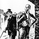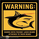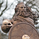8674 lượt xem
8674
RSX is a "noise free" version of RSI , with no added lag. This is a port of a clone to Pine.

Use this indicator just like RSI . I have set the default length to 14, feel free to experiment. You can reduce length with out worrying about jaggedness.

Options page has all the configurable parameters. You can use the plot names to setup alerts.

I have couple of more indicators built using this, will post them later. Let me know what you think about this indicator.

RSX Clone compared to normal RSI:

Smoothness helps a lot in identifying the divergences correctly:

http://www.jurikres.com/down__/product_g...

List of my public indicators: http://bit.ly/1LQaPK8
List of my app-store indicators: http://blog.tradingview.com/?p=970
//
// @author LazyBear
//
// List of my public indicators: http://bit.ly/1LQaPK8
// List of my app-store indicators: http://blog.tradingview.com/?p=970
//
//
study(title="JMA RSX Clone [LazyBear]", shorttitle="RSXC_LB", overlay=false)
src=input(close)
length=input(14)
lvlob = input(70, title="OB Level")
lvlos = input(30, title="OS Level")
mid  = input(50, title="Mid Level")
clampmax = 100
clampmin = 0
f90_ = (nz(f90_) == 0.0) ? 1.0 : (nz(f88) <= nz(f90_)) ? nz(f88)+1 : nz(f90_)+1
f88 = (nz(f90_) == 0.0) and (length-1 >= 5) ? length-1.0 : 5.0
f8 =  100.0*(src)
f18 = 3.0 / (length + 2.0)
f20 = 1.0 - f18
f10 = nz(f8)
v8 = f8 - f10
f28 = f20 * nz(f28) + f18 * v8
f30 = f18 * f28 + f20 * nz(f30)
vC = f28 * 1.5 - f30 * 0.5
f38 = f20 * nz(f38) + f18 * vC
f40 = f18 * f38 + f20 * nz(f40)
v10 = f38 * 1.5 - f40 * 0.5
f48 = f20 * nz(f48) + f18 * v10
f50 = f18 * f48 + f20 * nz(f50)
v14 = f48 * 1.5 - f50 * 0.5
f58 = f20 * nz(f58) + f18 * abs(v8)
f60 = f18 * f58 + f20 * nz(f60)
v18 = f58 * 1.5 - f60 * 0.5
f68 = f20 * nz(f68) + f18 * v18
f70 = f18 * f68 + f20 * nz(f70)
v1C = f68 * 1.5 - f70 * 0.5
f78 = f20 * nz(f78) + f18 * v1C
f80 = f18 * f78 + f20 * nz(f80)
v20 = f78 * 1.5 - f80 * 0.5
f0 = ((f88 >= f90_) and (f8 != f10)) ? 1.0  : 0.0
f90 = ((f88 == f90_) and (f0 == 0.0))  ? 0.0  : f90_
v4_ = ((f88 < f90) and (v20 > 0.0000000001)) ? (v14 / v20 + 1.0) * 50.0 : 50.0
rsx = (v4_ > 100.0) ? 100.0 : (v4_ < 0.0) ? 0.0 : v4_
hline(lvlob, color=red, title="OB Level"), hline(mid,linewidth=2, title="Mid Level"), hline(lvlos, color=green, title="OS Level"),
plot(rsx, color=red, linewidth=2, title="RSXC")Awesome, this is my favorite rsi indicator now
Phản hồiHello, I am interested in LB_RSRL but cant find anything similar in your list of indicators. Would PM code, please? Thanks
Phản hồiDanielKovacik
Hi Daniel,

RSI S/R level is part of one of my appstore packages. More info: https://www.tradingview.com/market/lb-srlevels/

Feel free to ping me if you need more info.
Phản hồiLazyBear
Have you given any thought to coding a Hilbert Sine Wave Clone?
Phản hồiDare2
In the pipeline, hopefully someday will get to that :)
Phản hồi